## Wednesday, March 9, 2016

### Method to compute consensus edge lengths (branch lengths for a consensus topology)

I just added a simple phytools function to compute consensus edge lengths - or, more accurately, edge lengths for a consensus topology - by various seemingly sensible methods, as follows:

Method 1: Compute the mean edge length for each edge in the consensus tree setting the length for each tree in which the edge is absent to zero. (Default setting. Function arguments `method="mean.edge"` and `if.absent="zero"`.)

Method 2: Compute the mean edge length, but ignore trees in which the edge is absent. (Function arguments `method="mean.edge"` and `if.absent="ignore"`.)

Method 3: Compute the non-negative least squares edge lengths on the consensus tree using the mean patristic distance matrix. (Function argument `method="least.squares"`.) If the input trees are rooted & ultrametric, this can be used to produce a consensus tree that is also ultrametric.

By default the consensus tree method used is majority rule consensus (e.g., `consensus(trees,p=0.5)`); however the user can supply their own consensus tree using the optional argument, logically, `consensus.tree`.

Code for this new function can be viewed on phytools GitHub page, here.

The following is a quick demo using a real empirical data set in which the tree tip labels have been changed:

``````trees
``````
``````## 512 phylogenetic trees
``````
``````## method 1
t1<-consensus.edges(trees)
plotTree(t1,fsize=0.4)
``````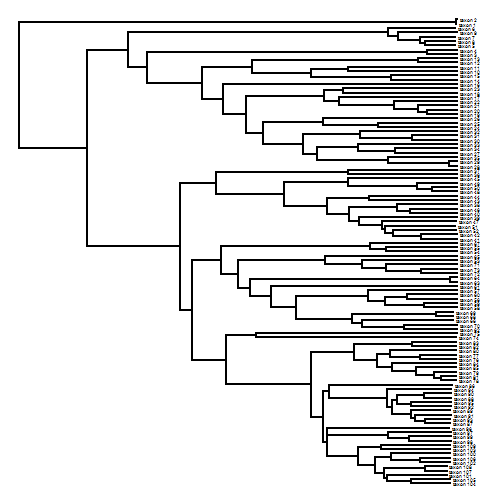``````## method 2
t2<-consensus.edges(trees,if.absent="ignore")
plotTree(t2,fsize=0.4)
``````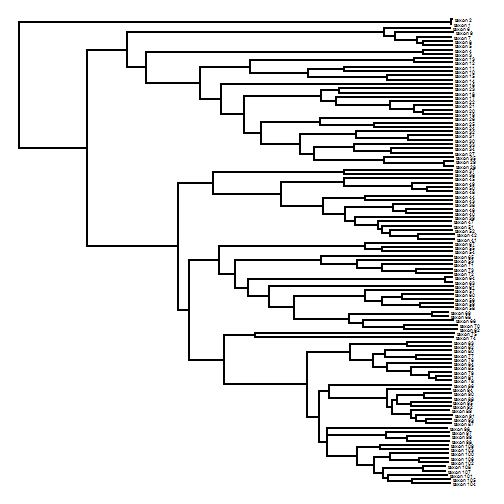``````## method 3
t3<-consensus.edges(trees,method="least.squares")
plotTree(t3,fsize=0.4)
``````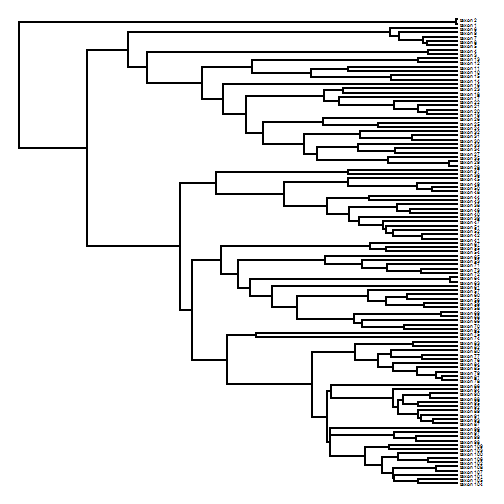``````## method 1, but with a 95% consensus tree
t1.p95<-consensus.edges(trees,consensus.tree=consensus(trees,p=0.95))
plotTree(t1.p95,fsize=0.4)
``````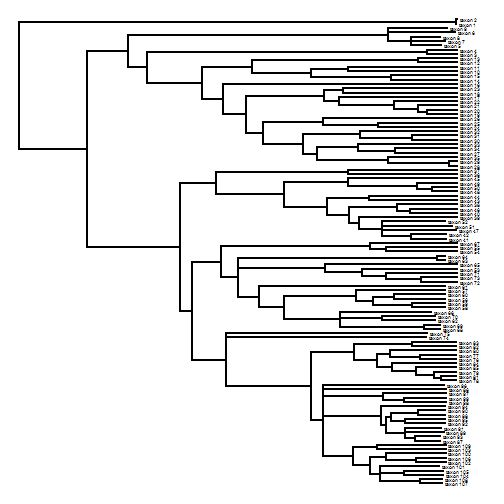It's hard to compare the results from each method, but we could, for instance, correlate the patristic distance matrices that each consensus tree implies:

``````tips<-t1\$tip.label
plot(cophenetic(t1)[tips,tips],cophenetic(t2)[tips,tips],
xlab="Method 1",ylab="Method 2")
h<-2*max(nodeHeights(t1))
lines(c(0,h),c(0,h),lty="dashed",col="red",lwd=3)
``````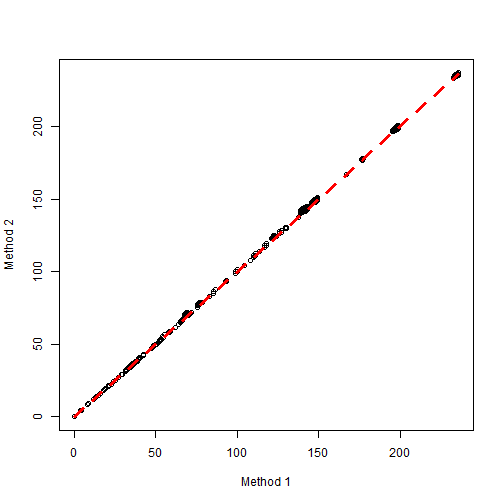``````plot(cophenetic(t1)[tips,tips],cophenetic(t3)[tips,tips],
xlab="Method 1",ylab="Method 3")
lines(c(0,h),c(0,h),lty="dashed",col="red",lwd=3)
``````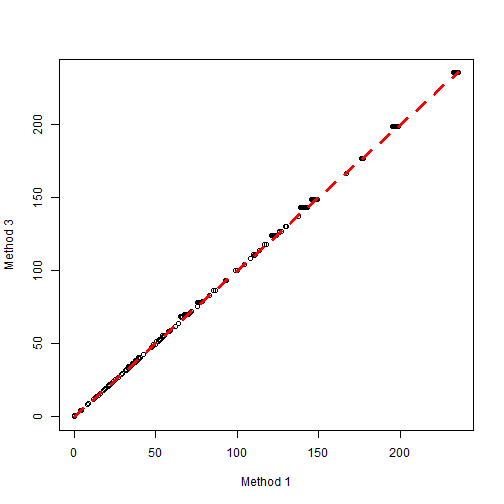…. you get the idea.

In this case, at least, it seems to matter relatively little which approach is chosen; however this is an empirical result, so that should not be generally assumed.

Note that this was developed for rooted trees; but it may apply to unrooted trees. I haven't checked yet.

Not sure what other methods are out there for computing consensus edge lengths, nor how easy they would be to add. This is not exactly my area of expertise.

1.This makes me wonder what method MrBayes uses when it gives a half-compat/majority-rule tree...

Speaking of which, I've see several methods for calculating half-compat trees from non-ultrametric Bayesian tip-dating analyses return negative branch-lengths when sampled ancestors are involved (which tend to be on terminal zero-length branches). Do you think any of the approaches you suggest would be sensitive to non-ultrametricity or zero-length branches?

1.Hi David. Yes, I think that what MrBayes sumt does is equivalent to Method 2 - based on skimming the docs only.

Do you end up with negative branch lengths as a consequence of the consensus method - or do the sampled trees have negative length edges?

2.To answer your question (sorry, it took a while), the trees themselves contain zero-length branches, and the consensus methods I've seen return negative branch lengths. Weird stuff.

2.Hi Liam, sorry if I can't find this, but is there any way to implement Pagel's Lambda in batches using multiPhylosignal, as you can with Blomberg's K? Thanks, Kevin

3.Hi Liam,

I was wondering if it would make sense to generate supertree(s) from partly overlapping trees, and then use one of these 3 methods to assign branch length on the resulting supertree(s).

The original trees would probably need to be ultrametric/time-calibrated for branch lengths to make sense in the resulting supertree, I think.

Would something like that be feasible as long as it makes sense? Thanks!

4.Hi Liam,

I used this function to create a consensus of the Erikson 10,000 trees pulled from BirdTree.org with a subset of 800 species. The system.time for the function was ~96 hours. I did tests for 5 species and 10 species which were ~55 seconds and ~180 seconds respectively. Why does the function take so long? Is there a way to parellelize it? Thanks!

Note: due to the very large amount of spam, all comments are now automatically submitted for moderation.